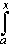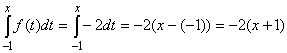#Interactive Real Analysis

Next | Previous | Glossary | Map

## 7.1. Riemann Integral

### Examples 7.1.21(c):

Show that if one starts with an integrable function f in the Fundamental Theorem of Calculus that is not continuous, the corresponding function F may not be differentiable.
Our Fundamental Theorem states that if we start with a continuous function f(t) over some interval [a, b] then the new function F(x) obtained by integrating f from a to some variable value x is differentiable:
F(x) =f(t) dt is differentiable as long as f is continuous
Now let's start with a simple step function that is integrable but not continuous over the interval, say, [-1, 1]. DefineThen for if x < 0 we have:and for x0But thenwhich is not differentiable at x = 0.
Next | Previous | Glossary | Map